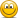Problems in GARP's 2020 FRM material

David Harper CFA FRM

David Harper CFA FRM
Staff member
Subscriber
@MilaBank agree but tiny nit: should be "USD 100.4375 (= 100 + (0.5 × 0.875))" to reflect one-half the 0.875 coupon. Thank you!

MilaBank

New Member
@MilaBank agree but tiny nit: should be "USD 100.4375 (= 100 + (0.5 × 0.875))" to reflect one-half the 0.875 coupon. Thank you!
yes, sorry, my typo nowevolutionest

New Member
Hi! I have a concern about QA chapter 9 answer to the question 9.4, which says that the probability of including a single irrelevant regressor is 1 - alpha, where alpha is the test size. But shouldn't it actually be alpha? As I am understanding this is that including the irrelevant regressor is incorrectly rejecting the true 0-hypothesis, which is a Type 1 error and the probability of that is equal to the test size. Or am I missing something?

Hi @lucaslim ,